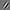# Visualization of optimal stimuli and invariances for Tiled Convolutional Neural Networks.

## Introduction

Visual inspection of trained neural networks can often provide intuition about what they are learning, and has been well-studied in the literature (e.g., see Erhan et al. ). This is typically done by visualizing the optimal stimuli for each hidden unit. However, the optimal stimulus of a hidden unit is just a single image, and does not give us much information on the range of inputs that the unit is invariant to. Hence, optimal stimuli alone often do not adequately characterize the behavior of complex hidden units.

To address this problem, Berkes and Wiskott  study networks in which the output of hidden neurons are quadratic functions of inputs, and describe a method of visualizing the invariant directions of a hidden unit, i.e., the directions in input space along which the activation of each hidden unit changes the least. By visualizing these directions, we can gain insight into the transformations that each hidden unit is invariant and robust to. Moreover, by visualizing the least invariant directions, i.e., those in which the activation of the hidden unit changes the most, we can discover what the hidden unit specifically codes for.

Here, we extend Berkes and Wiskott's approach to arbitrary networks. For each hidden unit, the idea is to find its optimal stimulus and then approximate its activation by a second-order Taylor expansion centered at the optimal stimulus. To do this, we numerically compute the Hessian at the optimal stimulus. Varying along the eigenvectors of this Hessian matrix then lets us generate videos of invariances for the hidden units of arbitrary networks. Analogously to , this approach is mathematically equivalent to finding the geodesics along the unit sphere along which the activation of the hidden unit drops the least in a local neighborhood around the optimal stimulus.

We demonstrate this technique on the Tiled Convolutional Neural Networks (TCNNs) described in , which are non-quadratic because of the squareroot activation in the second layer, and show that TCNNs do learn meaningful invariances.

## Algorithm Details

Further details about the algorithm we used can be found here.

visualization.tar.gz

The code can be used for general networks with any type of activation functions. We use Mark Schmidt's minConf  to carry out the required optimization routines, and expect the user to provide the activation function and its gradient with respect to the input pixels.

David Kamm also assisted in the development of this code. If you like the code, please cite:

Q.V. Le, J. Ngiam, Z. Chen, D. Chia, P. Koh, A.Y. Ng. Tiled Convolutional Neural Networks. NIPS, 2010. [latex bib entry]

## Visualization examples

Adobe Flash is required to view the animations in the following examples.

We present visualization examples from a 16-map, one-layered network trained on a grayscale version of the CIFAR-10 dataset . We picked one unit from the network, and visualized its optimal stimulus:As expected, it looks like a Gabor filter. Note that the optimal stimuli and invariance videos shown here are actually 10 pixels by 10 pixels, because our networks use local receptive fields; however, for ease of visualization, we show enlarged images and videos. While visualizing the optimal stimulus can be helpful, more intuition of what this particular hidden unit codes for can be obtained from visualizing what it is invariant to. For example, from the top five invariant directions for this one unit, we see three types of invariance:

 Translation invariance Rotational invariance Scale invariance

Moreover, visualizing its least invariant direction also yields an interesting result; it turns out that this particular hidden unit's activation changes the most when the edges of the Gabor filter rotate in opposite directions from each other:

This suggests that the unit is highly sensitive to well-formed lines whose edges are parallel.

## More visualizations

Here are some more visualizations of other hidden units from the same network:

Optimal stimulusInvariance visualizationThe invariance videos shown here are representative of the top invariance directions for most of the hidden units in our trained networks. This is not the case for hidden units whose weights are randomized (see  for a discussion). In the random case, while we might see units with grating-like optimal stimuli, their invariance directions are often messy and are not clean cases of any particular type of invariance, unlike the videos shown above. For example, this is a visualization of a typical unit:

Optimal stimulusInvariance visualizationThis illustrates the additional information that visualizing the invariant directions provides over just visualizing the optimal stimulus, and shows that our pretraining through topographic ICA results in hidden units learning more meaningful representations when compared with randomized hidden units.

## References

 Berkes, P. and Wiskott, L. On the analysis and interpretation of inhomogeneous quadratic forms as receptive fields. Neural Computation, 2006.

 Q.V. Le, J. Ngiam, Z. Chen, D. Chia, P. Koh, A.Y. Ng. Tiled Convolutional Neural Networks. NIPS, 2010.

 D. Erhan, Y. Bengio, A. Courville, and P. Vincent. Visualizing higher-layer features of a deep network. Technical report, University of Montreal, 2009.

 M. Schmidt, minConf, http://www.cs.ubc.ca/~schmidtm/Software/minConf.html , 2008.

 A. Krizhevsky. Learning multiple layers of features from tiny images. Technical report, U. Toronto, 2009.

 A. Saxe, P.W. Koh, Z. Chen, M. Bhand, B. Suresh, A. Ng. On random weights and unsupervised feature learning. NIPS Deep Learning and Unsupervised Feature Learning Workshop, 2010.